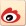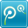# 2012广东公务员考试行测等差数列巧解分析

2012-03-05    来源：广东公务员考试网 字号： T | T | T我要提问
2012年广东公务员考试大纲暂未公布，根据广东公务员考试网（http://www.gdgkw.org/）专家对近年广东公务员考试分析数字推理试题难度正逐年加大，等差数列及其变式、幂数列已成为考试的热点，考生应重点掌握特别总结等差数列题目的解题方法，可结合2012年广东公务员考试综合教材来复习。希望考生攻克数字推理难关，踏上公务员之路。
等差数列的项和运算符号按某种规律排列所得算式的速算与巧算，这里有时要改变运算顺序，有时需通过裂项来实现求和。按照给定的法则进行定义新运算。较为复杂的整数四则运算问题，下面通过例题进行详细介绍。
1.计算：3333×5555+6×4444×2222.
=3×1111×5×1111+6×1111×4×2×1111=15×1111×1111+2×3×1111×1111×4×2
=1111×1111(15+48)=1111×1111×63=1111×1111×9×7
=9999×7777=(1000-1)×7777=77770000-7777=77762223
2.求和：l×2+2×3+3×4+…+9×10.
解：通过这个题，学“裂项”。看：
1×2=1×2×3÷3;2×3=2×3×3÷3=(2×3×4-1×2×3)÷3;
3×4=3×4×3÷3=(3×4×5-2×3×4)÷3……
可以发现：n×(n+1)×3÷3=[n×(n+1)×(n+2)-(n-1)×n×(n+1)]÷3
于是原式=(1×2×3+2×3×4-1×2×3+3×4×5-2×3×4+…+9×10×11-8×9×10)÷3
=9×10×11÷3=330
注意隔位抵消
3.两个十位数1111111111与9999999999的乘积中有几个数字是奇数?
解1：1111111111×9999999999
=1111111111×(10000000000-1)=11111111110000000000-1111111111=1111111118888888889
有10个数为奇数。
解2： 1×9 　 = 9 奇数的个数为1
11×99 = 1089 奇数的个数为2
111×999 =110889 奇数的个数为3
1111×9999 =11108889 奇数的个数为4
… …
11111111111×999999999=1111111110888888889 奇数的个数为10
显然其奇数的个数为10。
4.对于自然数1，2，3，…，100中的每一个数，把它非零数字相乘，得到100个乘积(例如23，积为2×3=6;如果一个数仅有一个非零数字，那么这个数就算作积，例如与100相应的积为1).问：这100个乘积之和为多少?
解：从1，2，…，9， 的乘积的数字和是45;
从11，12，…，19 的乘积的数字和是1×45;
从21，22， …，29， 的乘积的数字和是2×45，
…，
从91，92，…，99， 的数字和是9×45;
而10，20，…，90， 的数字和是45，
100的为1，故，其总和为：
(1+1+2+3+…+9+1)×45+1=47×45+1=2116

阅读了此文的人还阅读了
2012年广东省公务员考试资讯专题相关文章相关问题